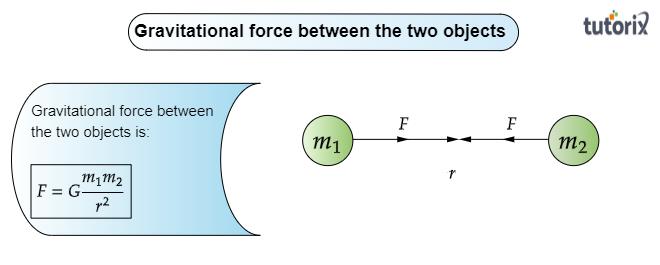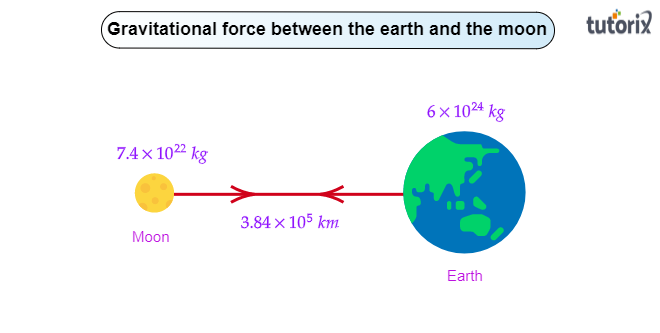# If the moon attracts the earth, why does the earth not move towards the moon?

To do:

To explain why the earth does not move towards the moon if the moon attracts the earth.

Solution:

To explain why the earth does not move toward the moon it is necessary to know about the nature of the gravitational force. Let us know a little about the force of gravitation:

Force of gravitation:

The universal law of gravitation states that every object exerts a gravitational force of attraction on every other object. It is true, but we don't notice such forces because here on the earth no object has an extremely large mass. Therefore, the gravitational forces between the two objects are so weak that they can't be noticed.

In a room, two objects don't move towards each other due to the gravitational force of attraction because this force is very weak as their masses are very small.

Formula for the gravitational force:

If the two bodies of masses $m_1$ and $m_2$ are kept at a distance $r$ from each other, then the gravitational force:

$F=G\frac{m_1m_2}{r^2}$

Here, $G$ is the gravitational constant. Its value is $6.67\times10^{-11}m^3kg^{-1}s^{-2}$.The earth does not move towards the moon :

Both moon and the earth experience equal gravitational forces from each other. As the mass of the earth$(around\ 81\ times\ that\ of\ the\ moon)$ is larger than that of the moon, so the rate of acceleration in earth toward the moon is lesser than that of the moon. So the earth does not move.Updated on: 10-Oct-2022

51 Views